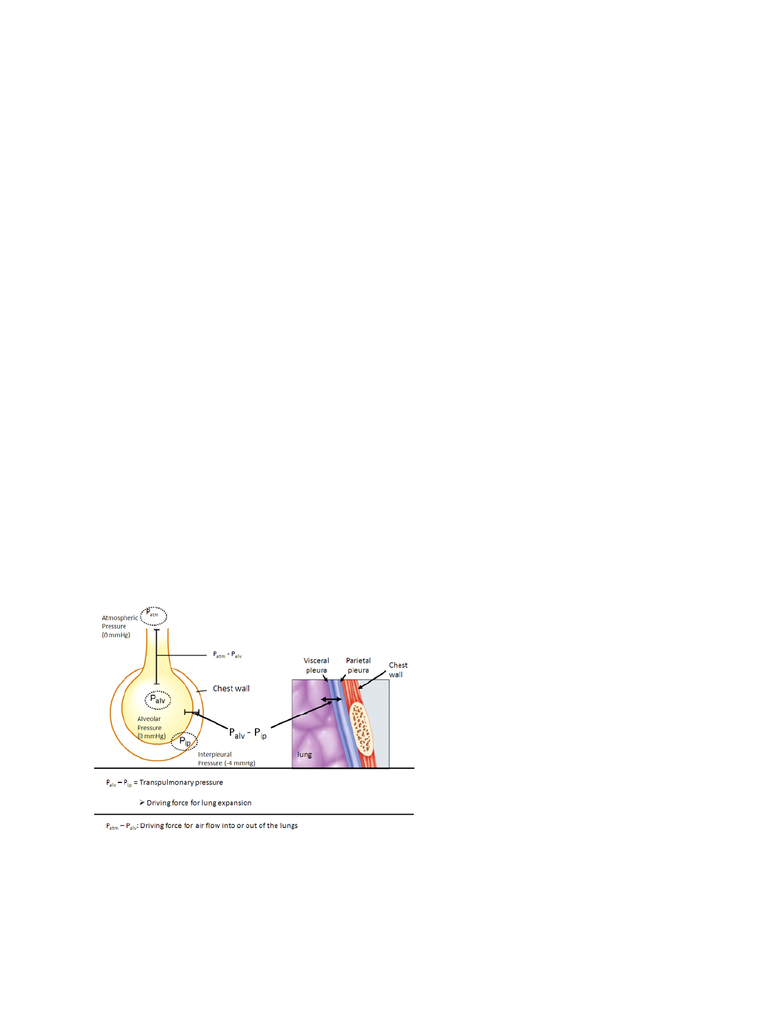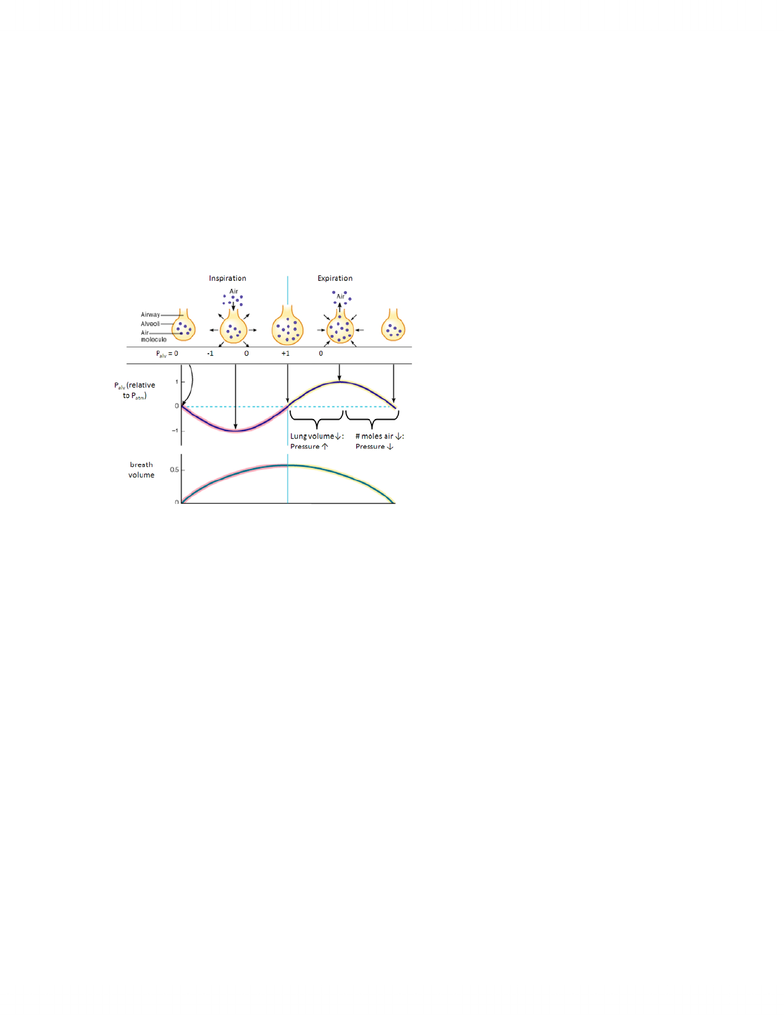# BIOC34H3 Lecture Notes - Lecture 10: Ideal Gas Law, Transpulmonary Pressure, Gas Constant

26 views5 pages
School
Course
Professor1
Lecture 10: Lung Mechanics (continued) and Spirometry
1. The Ideal Gas Law
The Ideal Gas Law is as follows:
PV = nRT
Where P is gas pressure, V is the volume in which the gas is contained, n is the number of moles
of gas, R is the universal gas constant, and T is temperature in degrees Kelvin. Pressure can be
calculated by the equation P = nRT/V. Boyle's Law, which states that for a given quantity of gas
in a chamber, the gas pressure is inversely proportional to the volume of the chamber.
There are three main pressures associated with pulmonary mechanics as well as two important
pressure differentials. Atmospheric pressure, inter-alveolar pressure, and inter-pleural
pressure are the three pressure values, and the important pressure differentials are between the
former two (which is the driving force for moving air in and out of the lungs) and between the
latter two (called transpulmonary pressure, which is the driving force for lung expansion).
Air flow in and out of the lungs is caused by differences between atmospheric pressure and
pressure inside the lungs (inter-alveolar pressure). If the former pressure is higher than the latter,
air will flow into the lungs, and vice versa. We can calculate air flow via this equation:
Air Flow = (atmospheric pressure – inter-alveolar pressure) / Resistance
We consider (in this case) atmospheric pressure to be constant (atmospheric pressure changes are
primarily due to changes in altitude).
Unlock document

This preview shows pages 1-2 of the document.
Unlock all 5 pages and 3 million more documents.2
2. Pressure and Volume Changes during Inspiration and Expiration
As the lung expands, inter-alveolar pressure will decrease. As inter-alveolar pressure falls lower
relative to atmospheric pressure, the lungs will begin to fill with molecules of air. As the lungs
fill, pressure begins to increase again, and when it reaches equilibrium with the pressure of air
outside the lungs, air flow will cease. Even though Palv relative to Patm goes down and up during
inspiration, breath volume increases during this entire time period until equilibrium is reached
(i.e., Palv relative to Patm is zero).
During expiration, as the lungs recoil to the “resting state”, inter-alveolar pressure increases
relative to atmospheric pressure forcing air out of the lungs. When enough air has been exhaled,
pressure will begin to decrease again, until it reaches equilibrium and the process begins again. If
one were to graph inter-alveolar pressure relative to atmospheric pressure during the process of a
breath, it would resemble a sine wave: a shallow dip down and a shallow curve up, and back
again.
Unlock document

This preview shows pages 1-2 of the document.
Unlock all 5 pages and 3 million more documents.

## Document Summary

Lecture 10: lung mechanics (continued) and spirometry: the ideal gas law. Where p is gas pressure, v is the volume in which the gas is contained, n is the number of moles of gas, r is the universal gas constant, and t is temperature in degrees kelvin. Pressure can be calculated by the equation p = nrt/v. Boyle"s law, which states that for a given quantity of gas in a chamber, the gas pressure is inversely proportional to the volume of the chamber. There are three main pressures associated with pulmonary mechanics as well as two important pressure differentials. Air flow in and out of the lungs is caused by differences between atmospheric pressure and pressure inside the lungs (inter-alveolar pressure). If the former pressure is higher than the latter, air will flow into the lungs, and vice versa. We can calculate air flow via this equation: Air flow = (atmospheric pressure inter-alveolar pressure) / resistance.

# Get access

\$10 USD/m
Billed \$120 USD annually
Homework Help
Class Notes
Textbook Notes